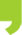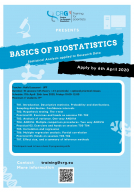## You are here

• You are here:
• Home > Events > Basics of Biostatistics 2020: Statistical Analysis applied to Research Data

# Basics of Biostatistics 2020: Statistical Analysis applied to Research Data

##Basics of Biostatistics 2020: Statistical Analysis applied to Research DataRegistration HERE

17/04/2020 26/06/2020

## Basics of Biostatistics 2020: Statistical Analysis applied to Research Data

Description:

The course will give an overview of important concepts and methods used to analyse “Biomedical data”. The emphasis will be on the understanding of statistical concepts and their interpretation in a research framework. After a general introduction on probability theory and statistical inference, an emphasis will be made on common statistical methods. Particular cases will be used as illustrative examples.

The course will be composed of face-to-face theoretical lectures combined with practical sessions and additional short homework assignment.

Teacher: Hafid Laayouni (UPF)

Duration: 10 sessions (total 20h)

Schedule:  17th/24th / 30th April | 8th/22nd /29th May | 5th/12th/19th/26th June (additional optional sessions (discussing and resolving exercises) will be scheduled for Thursdays 3-4pm).

Where: CRG Training centre (PRBB patio)

Maximum nº students: 19

Requirements: Participants need to have basic R programing skills

Application deadline: 6th of April 2020

Title Type Date from 10:00-12:00
Introduction. Descriptive statistics. Probability and distributions. Sampling distribution. Confidence intervals Theory 17th April 2020
Hypothesis testing. The t-test. Theory 24th April 2020
Exercises and Hands on sessions 1-2. Practicum 30th April 2020
Analysis of variance. One-way ANOVA Theory 8th May 2020
ANOVA. Multiple comparison procedures. Two-way ANOVA. Theory 22nd May 2020
Hands on sessions 3-4. Practicum 29th May 2020
Correlation and regression Theory 5th June 2020
Multiple regression analysis. Partial correlation. Theory 12th June 2020
Hands on sessions 7-8 Practicum 19th June 2020
Effect Size and a summary of inference methods. Theory 26th June 2020

At the end of the course, the students will be able to:

• Express a scientific question in a mathematical formulation possible to analyse with statistical method.
• To be  confident about sampling methods and their impact on the quality of the data.
• Think on the basis of experimental design: replication, randomization and stratification.
• Think about the appropriate statistical method given the data we have (dependent and/or independent variable, continuous or categorical variables).
• Have a deep understanding of the meaning and interpretation of a test statistic and how to construct own statistic given data.
• Have a correct understanding of key words in basic statistic: null hypothesis, alternative hypothesis, error type I, error type II, statistical power, observational study, experimental study, causation and correlation, sampling error, random error, systematic error, sampling distribution, p-value, statistical and practical significance, effect size, statistical power.
• Correct interpretation of the result of a statistical test and been aware of the limitations of the applied methods.